Refer to our Texas Go Math Grade 6 Answer Key Pdf to score good marks in the exams. Test yourself by practicing the problems from Texas Go Math Grade 6 Lesson 5.1 Answer Key Adding Integers with the Same Sign.

Modeling Sums of Integers with the Same Sign

You can use colored counters to add positive integers and to add negative integers.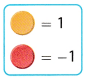Model with two-color counters.

(A) 3 + 4How many counters are there in total? __________
What is the sum and how do you find it?

(B) – 5 + (- 3)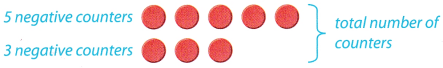How many counters are there in total? __________
Since the counters are negative integers, what is the sum? __________

Reflect

Question 1.
Communicate Mathematical Ideas When adding two numbers with the same sign, what sign do you use for the sum?
The sum of two positive numbers is positive number
The sum of two negative numbers is negative number.
So, when you add two numbers with the same sign the sum has exactly that sign

Question 2.
What If? Suppose the temperature is – 1°F and drops by 3°F. Explain how to use the number line to find the new temperature.
First graph number line with units.
Start at 0 and move 1 unit left to represent number – 1.
Then, start at – 1 and move 3 units left (because temperature drops by 3) and read new temperature from number line.

Graph: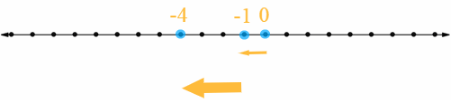New temperature would be – 4 because it is 4 units left from 0 on the number line.

Question 3.
Communicate Mathematical Ideas How would using a number line to find the sum 2 + 5 be different from using a number line to find the sum – 2 + (- 5)?
To find 2 + 5 on number line you start at 0, then move 2 units right and then move 5 units also right

To find – 2 + (- 5) on number line you start at 0, then move 2 units left and then move 5 units also left
So, only difference is where you move, left or right.

It is different direction of moving on number line.
For positive right, for negative left.

Question 4.
Analyze Relationships What are two other negative integers that have the same sum as – 2 and – 5?
First, graph number line with units.
Start at 0 and move 2 unit left to represent number – 2.
Then, start at – 2 and move 5 units left to add – 5 and read sum from number Line. It would be – 7.
So, it is 7 units left from 0, and you also could move 3 units left and then 4 units left and to be again 7 units left from 0.
Thus:
– 2 + (-5) = – 3 + (- 4)

Two other negative integers that have the same sum as – 2 and – 5 are -3 and -4.

Question 5.
Communicate Mathematical Ideas Does the Commutative Property of Addition apply when you add two negative integers? Explain.
It does.
You can apply Commutative Property when you add two negative integers because in both cases sum would be negative and equal minus sum of absolute values of that integers.

Example:
– 4 + (- 8) = – (|- 4| + |- 8|) = – (4 + 8)
– 8 + (- 4) = – (|- 8| + |- 4|) = – (8 + 4)
– (4 + 8) = – (8 + 4) → – 4 + (- 8) = – 8 + (- 4)

Question 6.
Critical Thinking Choose any two negative integers, Is the sum of the integers less than or greater than the value of either of the integers? Will this be true no matter which integers you choose? Explain.
The sum of two negative integers would always be less than the value of either of that integers.

This is because sum of two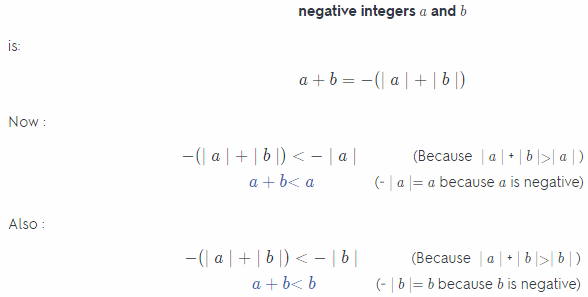The sum of two negative integers would always be less than the value of either of that integers.
Use a + b = – (|a| + |b|) for negative a and b.

Find each sum.

Question 7.
– 8 + (- 1) = ____________
First, find absolute values of given integers:
|- 8| = 8 and |- 1| = 1

Then, sum that absolute values:
8 + 1 = 9
Final result would be 9 or – 9, depending on sign of given integers (both positive then +, both negative then -).
Given integers are negative so final result is – 9.

Result is – 9.
Find absolute values, sum them and add minus if both were negative.

Question 8.
– 3 + (- 7) = __________
First find absolute values of given integer:
| – 3| = 3 and |- 7| = 7
Then, sum that absolute values:
3 + 7 = 10
Final result would be 10 or – 10, depending on sign of given integers (both positive then +, both negative then -).
Given integers are negative so final result is – 10.

Question 9.
– 48 + (- 12) = __________
First find absolute values of given integers:
|- 48| = 48 and |- 12| = 12
Then, sum that absolute values
48 + 12 = 60
Final result would be 60 or – 60, depending on sign of given integers (both positive then +, both negative then – ).
Given integers are negative so final result is – 60.

Question 10.
– 32 + (- 38) = _________
First find absolute values of given integers:
|- 32| = 32 and |- 38| = 38
Then, sum that absolute values
32 + 38 = 60
Final result would be 70 or – 70, depending on sign of given integers (both positive then +, both negative then – ).
Given integers are negative so final result is – 70.

Question 11.
109 + 191 = ___________
First find absolute values of given integers:
|109| = 109 and |191| = 191
Then, sum that absolute values
109 + 191 = 300
Final result would be 300 or – 300, depending on sign of given integers (both positive then +, both negative then – ).
Given integers are positive so final result is 300.
Note:
You could simply add them, but this is another (harder) way which shows that adding integers with the same sign like it was explained is correct

Question 12.
– 40 + (- 105) = ________
First find absolute values of given integers:
|- 40| = 40 and |- 105| = 105
Then, sum that absolute values
40 + 105 = 145
Final result would be 145 or – 145, depending on sign of given integers (both positive then +, both negative then – ).
Given integers are negative so final result is – 145.

Question 13.
– 150 + (- 1,500) = ________
First find absolute values of given integers:
|- 150| = 150 and |- 1,500| = 1,500
Then, sum that absolute values
150 + 1,500 = 1,650
Final result would be 1,650 or – 1,650 depending on sign of given integers (both positive then +, both negative then – ).
Given integers are negative so final result is – 1,650.

Question 14.
– 200 + (- 800) = _______
First find absolute values of given integers:
|- 200| = 200 and |- 800| = 800
Then, sum that absolute values
200 + 800 = 1,000
Final result would be 1,000 or – 1,000, depending on sign of given integers (both positive then +, both negative then – ).
Given integers are negative so final result is – 1,000.

Find each sum.

Question 1.
– 5 + (- 1)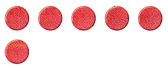In the first row there are 5 negative counters which represent – 5 and in second row there is 1 negative counter which represent – 1.
So, on the given picture there are 6 counters and all of them are negative.
(which represent – 6)
Thus, sum would be:
– 5 – (- 1) = – 6
On the picture there are 6 negative counters and thus – 5 + (- 1) = – 6.

a. How many counters are there? ________
6

b. Do the counters represent positive or negative numbers? ____________
negative

c. – 5 + (- 1) = _____________
– 6

Question 2.
– 2 + (- 7)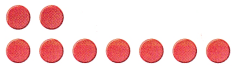In the first row there are 2 negative counters which represent – 2 and in second row there is 7 negative counter which represent – 7.
So, on the given picture there are 9 counters and all of them are negative.
(which represent – 9)
Thus, sum would be:
– 2 + (- 7) = – 9
On the picture there are 6 negative counters and thus – 2 + (- 7) = – 9.

a. How many counters are there? ________
9

b. Do the counters represent positive or negative numbers? ____________
negative

c. – 2 + (- 7) = _____________
– 9

Model each addition problem on the number line to find each sum.

Question 3.
– 5 + (- 2) = _____________First, graph number line with units.
Start at 0 and move 5 unit left to represent number – 5.
Then, start at – 5 and move 2 units left (to add – 2) and read sum from number line

Number line: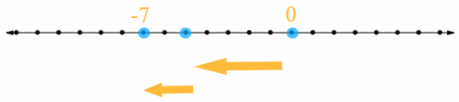Sum is – 7 because it is 7 units left from 0 on the number line.

Question 4.
– 1 + (- 3) = _____________First, graph number line with units.
Start at 0 and move 1 unit left to represent number – 1.
Then, start at – 1 and move 3 units left (to add – 3) and read sum from number line

Number line:Sum is – 4 because it is 4 units left from 0 on the number line.

Question 5.
– 3 + (- 7) = _____________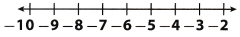First, graph number line with units.
Start at 0 and move 3 unit left to represent number – 3.
Then, start at – 3 and move 7 units left (to add – 7) and read sum from number line

Number line: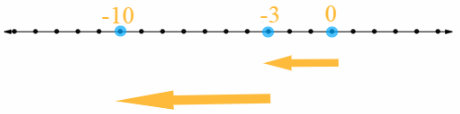Sum is – 10 because it is 10 units left from 0 on the number line.

Question 6.
– 4 + (- 1) = _____________First, graph number line with units.
Start at 0 and move 4 unit left to represent number – 4.
Then, start at – 4 and move 1 units left (to add – 1) and read sum from number line

Number line: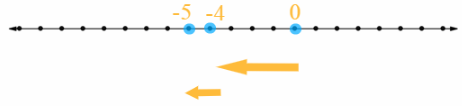Sum is – 5 because it is 5 units left from 0 on the number line.

Question 7.
– 2 + (- 2) = _____________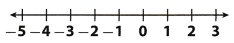First, graph number line with units.
Start at 0 and move 2 unit left to represent number – 2.
Then, start at – 2 and move 2 units left (to add – 2) and read sum from number line

Number line: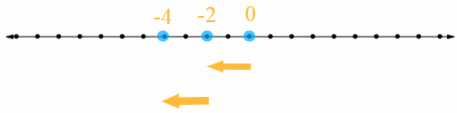Sum is – 4 because it is 4 units left from 0 on the number line.

Question 8.
– 6 + (- 8) = _____________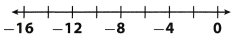First, graph number line with units.
Start at 0 and move 6 unit left to represent number – 6.
Then, start at – 6 and move 8 units left (to add – 8) and read sum from number line

Number line: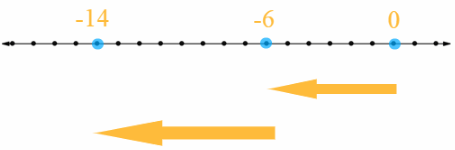Sum is – 14 because it is 14 units left from 0 on the number line.

Find each sum.

Question 9.
– 5 + (- 4) = _____________
First, find absolute values of given integers:
| – 5| = 5 and |- 4| = 4
Then, sum that absolute values:
5 + 4 = 9
Final result would be 9 or – 9, depending on sign of given integers (both positive then +, both negative then -).
Given integers are negative so final result is – 9.

Result is – 9
Find absolute values, sum them and add minus if both were negative.

Question 10.
– 1 + (- 10) = __________________
First, find absolute values of given integers:
| – 1| = 1 and |- 10| = 10
Then, sum that absolute values:
1 + 10 = 11
Final result would be 11 or – 11, depending on sign of given integers (both positive then +, both negative then -).
Given integers are negative so final result is – 11.

Result is – 11
Find absolute values, sum them and add minus if both were negative.

Question 11.
– 9 + (- 1) = ____________________
First, find absolute values of given integers:
| – 9| = 9 and |- 1| = 1
Then, sum that absolute values:
9 + 1 = 10
Final result would be 10 or – 10, depending on sign of given integers (both positive then +, both negative then -).
Given integers are negative so final result is – 10.

Result is – 10
Find absolute values, sum them and add minus if both were integers were negative.

Question 12.
– 90 + (- 20) = __________________
First, find absolute values of given integers:
| – 90| = 90 and |- 20| = 20
Then, sum that absolute values:
90 + 20 = 110
Final result would be 110 or – 110, depending on sign of given integers (both positive then +, both negative then -).
Given integers are negative so final result is – 110.

Result is – 110
Find absolute values, sum them and add minus if both were integers were negative.

Question 13.
– 52 + (- 48) = __________________
First, find absolute values of given integers:
| – 52| = 52 and |- 48| = 48
Then, sum that absolute values:
52 + 48 = 100
Final result would be 100 or – 100, depending on sign of given integers (both positive then +, both negative then -).
Given integers are negative so final result is – 100.

Result is – 100
Find absolute values, sum them and add minus if both were integers were negative.

Question 14.
5 + 198 = __________________
First, find absolute values of given integers:
| 5 | = 5 and |198| = 198
Then, sum that absolute values:
5 + 198 = 203
Final result would be 203 or – 203, depending on sign of given integers (both positive then +, both negative then -).
Given integers are negative so final result is 203.

Note: You could simply add them, but this is another way.

Result is – 203
Find absolute values, sum them and add minus if both were integers were negative.

Question 15.
– 4 + (- 5) + (- 6) = _____________
First, find absolute values of given integers:
|- 4| = 4 |- 5| = 5 and | – 6| = 6
Then, sum that absolute values:
4 + 5 + 6 = 15
Final result would be 15 or – 15, depending on sign of given integers (all positive then +, all negative then -).
Given integers are negative so final result is – 15.

Result is – 15
Find absolute values, sum them and add minus if given integers were negative.

Question 16.
– 50 + (- 175) + (- 345) = __________________
First, find absolute values of given integers:
|- 50| = 50 |- 175| = 175 and | – 345| = 345
Then, sum that absolute values:
50 + 175 + 345 = 570
Final result would be 570 or – 570, depending on sign of given integers (all positive then +, all negative then -).
Given integers are negative so final result is – 570.

Result is – 570
Find absolute values, sum them and add minus if given integers were negative.

Essential Question Check-In

Question 17.
How do you add integers with the same sign?
First, find absolute values of given integers;
Then, sum that absolute values;
If given integers were negative add minus.

Find absolute values, sum them and add minus if given integers were negative.

Question 18.
Represent Real-World Problems Jane and Sarah both dive down from the surface of a pool. Jane first dives down 5 feet, and then dives down 3 more feet. Sarah first dives down 3 feet, and then dives down 5 more feet.

a. Multiple Representations Use the number line to model the equation
– 5 + (- 3) = – 3 + (- 5).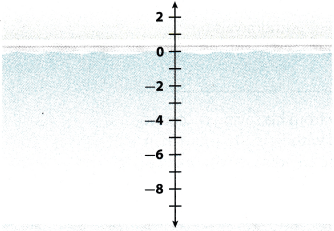First graph number Line with units.
Representing – 5 + (- 3):
Start at 0 and move 5 unit left to represent number – 5.
Then, start at – 5 and move 3 units left (to add – 3).
Now, you are 8 units Left from 0 on the number Line.
Representing – 3 + (- 5):
Start at 0 and move 3 unit left to represent number – 3.
Then, start at – 3 and move 5 units left (to add – 5).
Again, you are 8 units left from 0 on the number line.
So, it is equal.

Number line: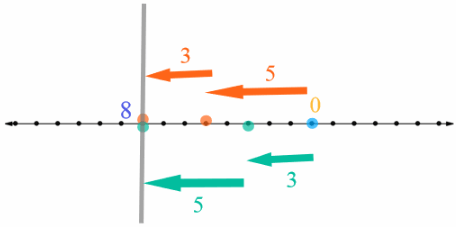b. Does the order in which you add two integers with the same sign affect the sum? Explain.
The order does not affect the sum because you move on number line in the same direction and get to the same value in both cases.

Question 19.
A golfer has the following scores for a 4-day tournament.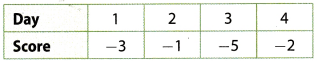What was the golfer’s total score for the tournament?
Total score equals:
– 3 + (- 1) + (- 5) + (- 2)
First find absolute values of given integers:
|- 3| = 3
|- 1| = 1
|- 5| = 5
|- 2| = 2
Then, sum that absolute values:
3 + 1 + 5 + 2 = 11
Final result would be 11 or – 11, depending on sign of given integers (both positive then +, both negative then -).
Given integers are negative so final result is – 11.

The golfer’s total score was – 11.
Find absolute values, sum them and add minus if all integers were negative.

Question 20.
A football team loses 3 yards on one play and 6 yards on another play. Write a sum of negative integers to represent this situation. Find the sum and explain how it is related to the problem.
Let represent the team’s score:
(-3) + (-6)
First, find absolute vaLues of given integers:
|- 3| = 3 and |- 6| = 6
Then, sum that absolute values
3 + 6 = 9
Final result would be 9 or – 9, depending on sign of given integers (both positive then +, both negative then -).
Given integers are negative so final result is – 9.
That means that the team loses 9 yards on two plays

Sum is – 9.
Find absolute values, sum them and add minus if both integers were negative.
That means that the team loses 9 yards on two plays.

Question 21.
When the quarterback is sacked, the team loses yards. In one game, the quarterback was sacked four times. What was the total sack yardage?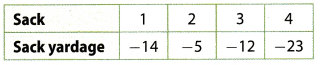The total sack yardage equals:
– 14 + (- 5) + (- 12) + (- 23)
First find absolute values of given integers:
|- 14| = 14
|- 5| = 5
|- 12| = 12
|- 23| = 23
Then, sum that absolute values
14 + 5 + 12 + 23 = 54
Final result would be 54 or – 54, depending on sign of given integers (both positive then +, both negative then -).
Given integers are negative so final result is – 54.

The total sack yardage was – 54.
Find absolute values, sum them and add minus if all integers were negative.

Question 22.
Multistep The temperature in Jonestown and Cooperville was the same at 1:00. By 2:00, the temperature in Jonestown dropped 10 degrees, and the temperature in Cooperville dropped 6 degrees. By 3:00, the temperature in Jonestown dropped 8 more degrees, and the temperature in Cooperville dropped 2 more degrees.

a. Write an equation that models the change to the temperature in Jonestown since 1:00.
Let calculate the change to the temperature in Jonestown:
(- 10) + (- 8)
First find absolute values of given integers:
|- 10| =10 and |- 8| = 8
Then, sum that absolute values
10 + 8 = 18
Final result would be 18 or – 18, depending on sign of given integers (both positive then +, both negative then -).
Given integers are negative so final result is – 18.
The temperature in Jonestown dropped 18 degrees since 1:00.

b. Write an equation that models the change to the temperature in Cooperville since 1:00.
Let calculate the change to the temperature in Cooperville:
(- 6) + (- 2)
First, find absolute values of given integers:
|- 6| = 6 and |- 2| = 2
Then, sum that absolute values
6 + 2 = 8
Final result would be – 8 or 8, depending on sign of given integers (both positive then +, both negative then -).
Given integers are negative so final result is – 8.
The temperature in Cooperville dropped 8 degrees since 1:00.

c. Where is it colder at 3:00, Jonestown or Cooperville?
Since the temperature was the same in both cities at 1:00, Jonestown is colder at 3:00, because – 18 is less than – 8.

Question 23.
Represent Real-World Problems Julio is playing a trivia game. On his first turn, he lost 100 points. On his second turn, he lost 75 points. On his third turn, he lost 85 points. Write a sum of three negative integers that models the change to Julio’s score after his first three turns.
Show how the score changes after three turns:
– 100 + (- 75) + (- 85)
First, find absoLute vaLues of given integers:
|- 100| = 100
|- 75| = 75
|- 85| = 85
Then, sum that absolute values
100 + 75 + 85 = 260
Final result would be 260 or – 260, depending on sign of given integers (both positive then +, both negative then -).
Given integers are negative so final result is – 260
Julio’s score got – 260 points after three turns.

The sum is
– 100 + (- 75) + (- 85)
and the change to his score is – 260.
Find absolute values, sum them and add minus if all integers were negative.

H.O.T. Focus on Higher Order Thinking

Question 24.
Multistep On Monday, Jan made withdrawals of $25,$45, and $75 from her savings account. On the same day, her twin sister Julie made withdrawals of$35, $55, and$65 from her savings account.

a. Write a sum of negative integers to show Jan’s withdrawals on Monday. Find the total amount Jan withdrew.
Let calculate Jan’s withdrawals:
– 25 + (- 45) + (- 75)
First find absolute values of given integers:
|- 25| = 25
|- 45| = 45
|- 75| = 75
then, sum that absoLute vaLues:
25 + 45 + 75 = 145
Final resuLt would be 145 or – 145, depending on sign of given integers (both positive then +, both negative then -).
Given integers are negative so final result is – 145
Jan withdrew $145. b. Write a sum of negative integers to show Julie’s withdrawals on Monday. Find the total amount Julie withdrew. Answer: Let calculate Julie’s withdrawals: – 35 + (- 55) + (- 65) First find absolute values of given integers: |- 35| = 35 |- 55| = 55 |- 65| = 65 Then, sum that absolute values 35 + 55 + 65 = 155 Final result would be 155 or – 155, depending on sign of given integers (both positive then +, both negative then -). Given integers are negative so final result is – 155. Julie withdrew$155.

c. Julie and Jan’s brother also withdrew money from his savings account on Monday. He made three withdrawals and withdrew $10 more than Julie did. What are three possible amounts he could have withdrawn? Answer: Their brother also made three withdrawals, and his total amount is$ 10 bigger then Julies.
He could have withdrawn first amount $10 bigger. Let calculate the first with drawal: – 35 + (- 10) First, find absolute values of given integers : |- 35| = 35 |- 10| = 10 Then, sum that absolute values: 35 + 10 = 45 Final result would be 45 or – 45, depending on sign of given integers (both positive then +. both negative then -). Given integers are negative so final result is – 45. He could have withdrawn amounts$ 45, $55 and$65.

Question 25.
Communicate Mathematical Ideas Why might you want to use the Commutative Property to change the order of the integers in the following sum before adding?
– 80 + (- 173) + (- 20)
First write down the sum:
– 80 + (- 173) + (- 20)
You might want to use the Commutative Property before adding because it makes adding easier
If you change the order of the integers – 173 and – 20, you will get the sum:
– 80 + (- 20) + (- 173)
Let calculate the sum
– 80 + (- 20)
First find absolute values of given integers:
|- 80| = 80 and |- 20| = 20
Then, sum that absoLute values
80 + 20 = 100
Final result would be 100 or – 100, depending on sign of given integers (both positive then +, both negative then -).
Given integers are negative so final result is – 100.
Now it is easy to calculate
– 100 + (- 173)

Using the Commutative Property to change the order of second and third integer before adding makes adding easier.

Question 26.
Critique Reasoning The absolute value of the sum of two different integers with the same sign is 8. Pat says there are three pairs of integers that match this description. Do you agree? Explain.
First pair of negative integers is – 1 and 7.
Find absolute values of given integers:
|- 1| = 1 and |- 7| = 7
Sum that absolute values:
1 + 7 = 8
The absolute value of the sum is 8.
Second pair is – 2 and – 6.
Find absolute values of given integers:
|- 2| = 2 and |- 6| = 6
Sum that absolute values:
2 + 6 = 8
The absolute value of the sum is 8.
Third pair is – 3 and – 5.
Find absolute values of given integers:
– 3 = 3 and – 5 = 5
Sum that absolute values:
3 + 5 = 8
The absolute value of the sum is 8.

First pair of positive integers is 1 and 7.
Find absolute values of given integers:
|1| = 1 and |7| = 7
Sum that absolute values:
1 + 7 = 8
The absolute value of the sum is 8
Second pair is 2 and 6.
Find absolute values of given integers:
|2| = 2 and |6| = 6
Sum that absolute values
2 + 6 = 8
The absolute value of the sum ¡s 8
Third pair is 3 and 5.
Find absolute values of given integers:
|3| = 3 and |5| = 5
Sum that absolute values:
3 + 5 = 8
The absolute value of the sum is 8.

There are six pairs of integers with the same sign: – 1 and – 7, – 2 and – 6, – 3 and – 5, 1 and 7, 2 and 6, 3 and 5.

Scroll to Top